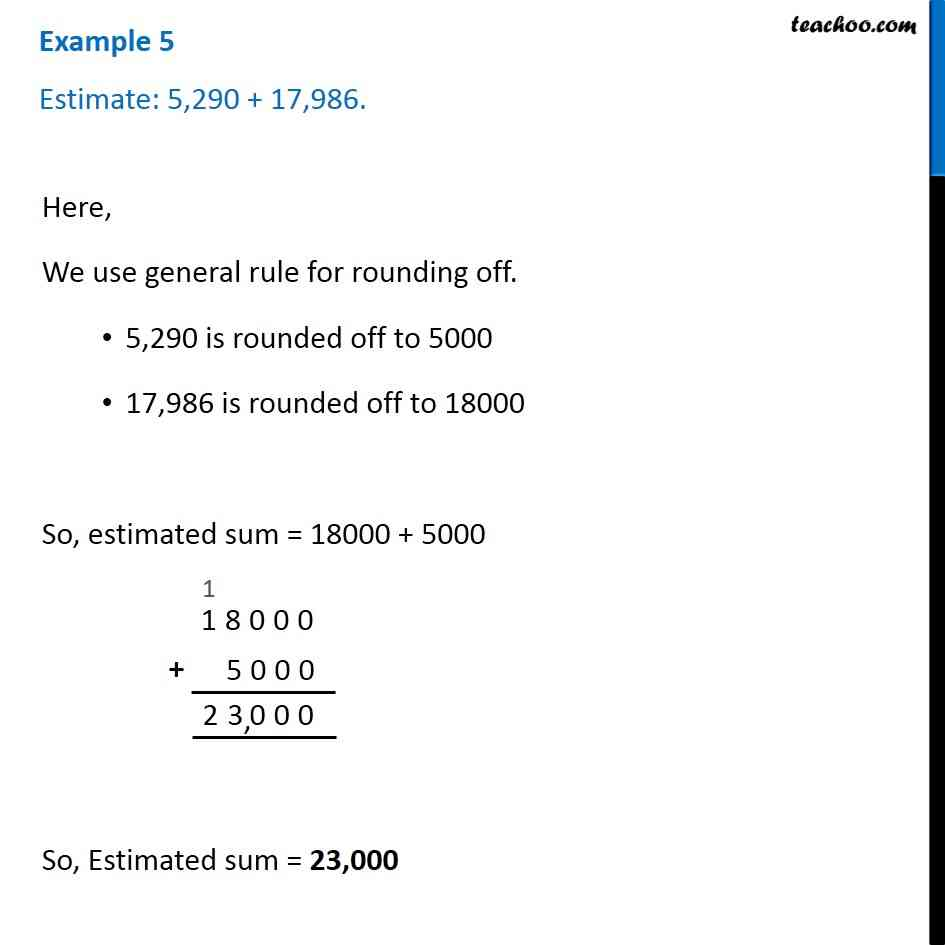1. Chapter 1 Class 6 Knowing our Numbers
2. Concept wise
3. Estimating sum and difference

Transcript

Example 5 Estimate: 5,290 + 17,986.Here, We use general rule for rounding off. 5,290 is rounded off to 5000 17,986 is rounded off to 18000 So, estimated sum = 18000 + 5000 So, Estimated sum = 23,000